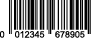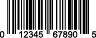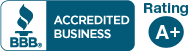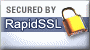# Check Digit Calculator

5-step algorithm for check digit calculation:

Let's assume that we are using the fictitious EAN Code 054321223455.
1. Add all of the digits in the odd positions (digits in position 1, 3, 5, 7, 9, and 11).
0 + 4 + 2 + 2 + 3 + 5 = 16
2. Add all of the digits in even positions (digits in position 2, 4, 6, 8, 10 and 12).
5 + 3 + 1 + 2 + 4 + 5 = 20
3. Multiply by 3.
20 * 3 = 60
4. Sum the results of steps 3 and 2.
16 + 60 = 76
5. Determine what number needs to be added to the result of step 4 in order to create a multiple of 10.
76 + 4 = 80
The check digit therefore equals 4.

EAN (GTIN-13) bar code check digit calculator
Enter or paste 12 digit codeDo you need professional print-ready digital EAN barcode graphics created? Please click here.

UPC (GTIN-12) bar code check digit calculator
Enter or paste 11 digit UPCDo you need professional print-ready digital UPC barcode graphics created? Please click here.
If you need assistance creating check digits in bulk (from a database or an Excel file, for example) we can help! We can create check digits for just about any type of code (EAN, UPC, SCC-14/GTIN-14, SSCC-18, and many more).